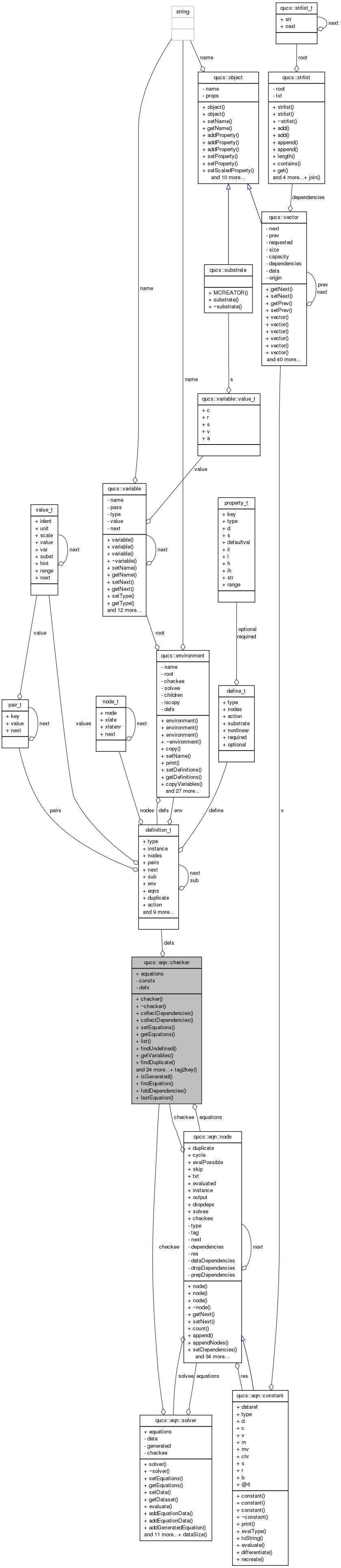Qucs-core  0.0.19
qucs::eqn::checker Class Reference

`#include <equation.h>`

Collaboration diagram for qucs::eqn::checker:[legend]

## Public Member Functions

checker ()
~checker ()
void collectDependencies (void)
void collectDependencies (node *)
void setEquations (node *)
nodegetEquations (void)
void list (void)
int findUndefined (int)
strlist * getVariables (void)
int findDuplicate (void)
int detectCycles (void)
nodeappendEquation (node *, node *)
void dropEquation (node *)
void reorderEquations (void)
int applyTypes (void)
int checkExport (void)
void constants (void)
int check (int noundefined=1)
strlist * variables (void)
nodeaddDouble (const char *, const char *, nr_double_t)
nodecreateDouble (const char *, const char *, nr_double_t)
nodeaddComplex (const char *, const char *, nr_complex_t)
nodecreateComplex (const char *, const char *, nr_complex_t)
nodeaddReference (const char *, const char *, char *)
nodecreateReference (const char *, const char *, char *)
void appendEquation (node *)
void addEquation (node *)
nodefindEquation (const char *const) const
bool containsVariable (const char *const) const
nr_double_t getDouble (const char *const) const
void setDouble (const char *const, nr_double_t)
qucs::vector getVector (const char *const) const
void setDefinitions (struct definition_t *d)
struct definition_tgetDefinitions (void)
nodefindProperty (char *)

## Static Public Member Functions

static const char * tag2key (int)
static int isGenerated (char *)
static nodefindEquation (node *, const char *const)
static strlist * foldDependencies (strlist *)
static nodelastEquation (node *)

nodeequations

## Private Attributes

bool consts
struct definition_tdefs

## Detailed Description

Definition at line 270 of file equation.h.

## Constructor & Destructor Documentation

 qucs::checker::checker ( )

Definition at line 1384 of file equation.cpp.

 qucs::checker::~checker ( )

Definition at line 1392 of file equation.cpp.

## Member Function Documentation

 node * qucs::checker::addComplex ( const char * type, const char * ident, nr_complex_t value )

Definition at line 2510 of file equation.cpp.

 node * qucs::checker::addDouble ( const char * type, const char * ident, nr_double_t value )

Definition at line 2500 of file equation.cpp.

 void qucs::checker::addEquation ( node * eqn )

Definition at line 2519 of file equation.cpp.

 node * qucs::checker::addReference ( const char * type, const char * ident, char * value )

Definition at line 2490 of file equation.cpp.

 node * qucs::checker::appendEquation ( node * root, node * last )

Definition at line 1742 of file equation.cpp.

 void qucs::checker::appendEquation ( node * eqn )

Definition at line 2526 of file equation.cpp.

 int qucs::checker::applyTypes ( void )

Definition at line 1833 of file equation.cpp.

 int qucs::checker::check ( int noundefined = `1` )

Definition at line 1855 of file equation.cpp.

 int qucs::checker::checkExport ( void )

Definition at line 1429 of file equation.cpp.

 void qucs::checker::collectDependencies ( void )

Definition at line 1409 of file equation.cpp.

 void qucs::checker::collectDependencies ( node * eqn )

Definition at line 1418 of file equation.cpp.

 void qucs::checker::constants ( void )

Definition at line 2472 of file equation.cpp.

 bool qucs::checker::containsVariable ( const char * const ident ) const

Definition at line 2443 of file equation.cpp.

 node * qucs::checker::createComplex ( const char * type, const char * ident, nr_complex_t value )

Definition at line 2557 of file equation.cpp.

 node * qucs::checker::createDouble ( const char * type, const char * ident, nr_double_t value )

Definition at line 2538 of file equation.cpp.

 node * qucs::checker::createReference ( const char * type, const char * ident, char * value )

Definition at line 2576 of file equation.cpp.

 int qucs::checker::detectCycles ( void )

Definition at line 1702 of file equation.cpp.

 void qucs::checker::dropEquation ( node * eqn )

Definition at line 1763 of file equation.cpp.

 int qucs::checker::findDuplicate ( void )

Definition at line 1642 of file equation.cpp.

 node * qucs::checker::findEquation ( node * root, const char * const n ) ` [static]`

Definition at line 1679 of file equation.cpp.

 node * qucs::checker::findEquation ( const char * const n ) const

Definition at line 1691 of file equation.cpp.

 node * qucs::checker::findProperty ( char * var )

Definition at line 1571 of file equation.cpp.

 int qucs::checker::findUndefined ( int noundefined )

Definition at line 1522 of file equation.cpp.

 strlist * qucs::checker::foldDependencies ( strlist * deps ) ` [static]`

Definition at line 1729 of file equation.cpp.

 struct definition_t* qucs::eqn::checker::getDefinitions ( void ) ` [inline, read]`

Definition at line 312 of file equation.h.

 nr_double_t qucs::checker::getDouble ( const char * const ident ) const

Definition at line 2596 of file equation.cpp.

 node* qucs::eqn::checker::getEquations ( void ) ` [inline]`

Definition at line 278 of file equation.h.

 strlist * qucs::checker::getVariables ( void )

Definition at line 1629 of file equation.cpp.

 qucs::vector qucs::checker::getVector ( const char * const ident ) const

Definition at line 2628 of file equation.cpp.

 int qucs::checker::isGenerated ( char * var ) ` [static]`

Definition at line 1505 of file equation.cpp.

 node * qucs::checker::lastEquation ( node * root ) ` [static]`

Definition at line 1755 of file equation.cpp.

 void qucs::checker::list ( void )

Definition at line 1482 of file equation.cpp.

 void qucs::checker::reorderEquations ( void )

Definition at line 1780 of file equation.cpp.

 void qucs::eqn::checker::setDefinitions ( struct definition_t * d ) ` [inline]`

Definition at line 311 of file equation.h.

 void qucs::checker::setDouble ( const char * const ident, nr_double_t val )

Definition at line 2610 of file equation.cpp.

 void qucs::checker::setEquations ( node * eqns )

Definition at line 1822 of file equation.cpp.

 const char * checker::tag2key ( int tag ) ` [static]`

Definition at line 1050 of file applications.h.

 strlist * qucs::checker::variables ( void )

Definition at line 2432 of file equation.cpp.

## Field Documentation

 bool qucs::eqn::checker::consts` [private]`

Definition at line 319 of file equation.h.

 struct definition_t* qucs::eqn::checker::defs` [private]`

Definition at line 320 of file equation.h.

Definition at line 316 of file equation.h.

The documentation for this class was generated from the following files: